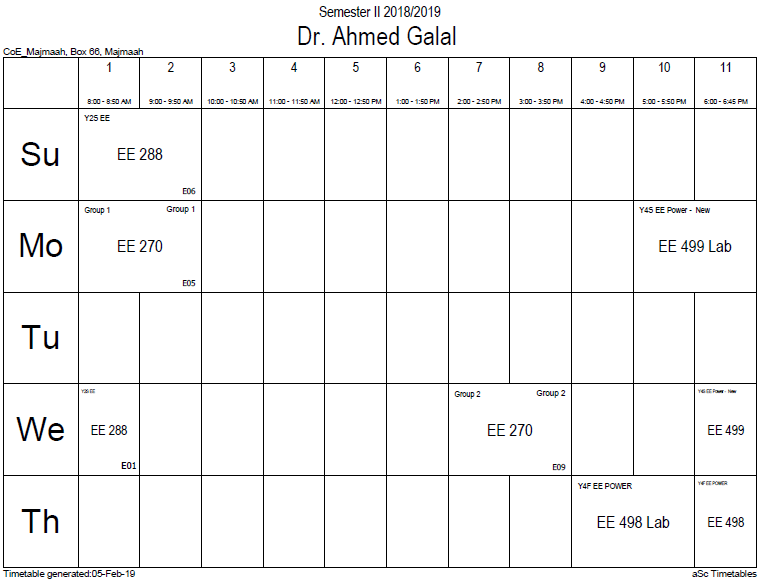## Nonlinear dynamo t

The kinematic approximation becomes invalid when the magnetic field becomes strong enough to affect the fluid motions. In that case the velocity field becomes affected by the Lorentz force, and so the induction equation is no longer linear in the magnetic field. In most cases this leads to a quenching of the amplitude of the dynamo. Such dynamos are sometimes also referred to as hydromagnetic dynamos. Virtually all dynamos in astrophysics and geophysics are hydromagnetic dynamos.

Numerical models are used to simulate fully nonlinear dynamos. A minimum of 5 equations are needed. They are as follows. The induction equation, see above. Maxwell's equation:$abla cdot mathbf{B}=0$

The (sometimes) Boussinesq conservation of mass:$abla cdot mathbf{u} = 0$

The (sometimes) Boussinesq conservation of momentum, also known as the Navier-Stokes equation:$frac{Dmathbf{u}}{Dt} = - abla p + u abla^2 mathbf{u} + ho^'mathbf{g} + 2mathbf{Omega} imes mathbf{u} + mathbf{Omega} imes mathbf{Omega} imes mathbf{R} + mathbf{J} imes mathbf{B}$

where$u$ is the kinematic viscosity,$ho^'$ is the density perturbation that provides buoyancy (for thermal convection$ho^'=alphaDelta T$,$Omega$ is the rotation rate of the Earth, and$mathbf{J}$ is the electrical current density.

Finally, a transport equation, usually of heat (sometimes of light element concentration):$frac{partial T}{partial t} = kappa abla^2 T +epsilon$

where T is temperature,$kappa=k/ ho c_p$ is the thermal diffusivity with k thermal conductivity,$c_p$ heat capacity, and$ho$ density, and$epsilon$ is an optional heat source. Often the pressure is the dynamic pressure, with the hydrostatic pressure and centripetal potential removed. These equations are then non-dimensionalized, introducing the non-dimensional parameters,$Ra=frac{galpha T D^3}{ u kappa} , E=frac{ u}{Omega D^2} , Pr=frac{ u}{kappa} , Pm=frac{ u}{eta}$

where Ra is the Rayleigh number, E the Ekman number, Pr and Pm the Prandtl and magnetic Prandtl number. Magnetic field scaling is often in Elsasser number units$B= ho Omega/sigma$.

### Office HoursNo office hours### My Timetable### Contactsemail: [email protected]

Phone: 2570

### Welcome

Welcome To Faculty of Engineering### IEEEhttp://www.ieee.org/

/

### Bookmarkshttp://www.utk.edu/research/

http://science.doe.gov/grants/index.asp

http://www1.eere.energy.gov/vehiclesandfuels/

http://www.eere.energy.gov/

### Upcoming Conferences### Engineering quotes### Travel Web Siteshttp://www.hotels.com/

http://www.orbitz.com/

http://www.hotwire.com/us/index.jsp

http://www.kayak.com/

### Blackboardستقام اختبارات الميدتيرم يوم الثلاثاء 26-6-1440

حسب الجدول المعلن بلوحات الاعلان

### Summer trainingThe registration for summer training will start from 5th week of second semesterClass registration week 1

### برنامج التجسير### إحصائية الموقع

عدد الصفحات: 2879

البحوث والمحاضرات: 1280

الزيارات: 100997